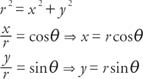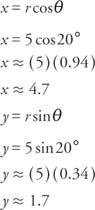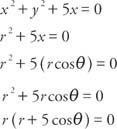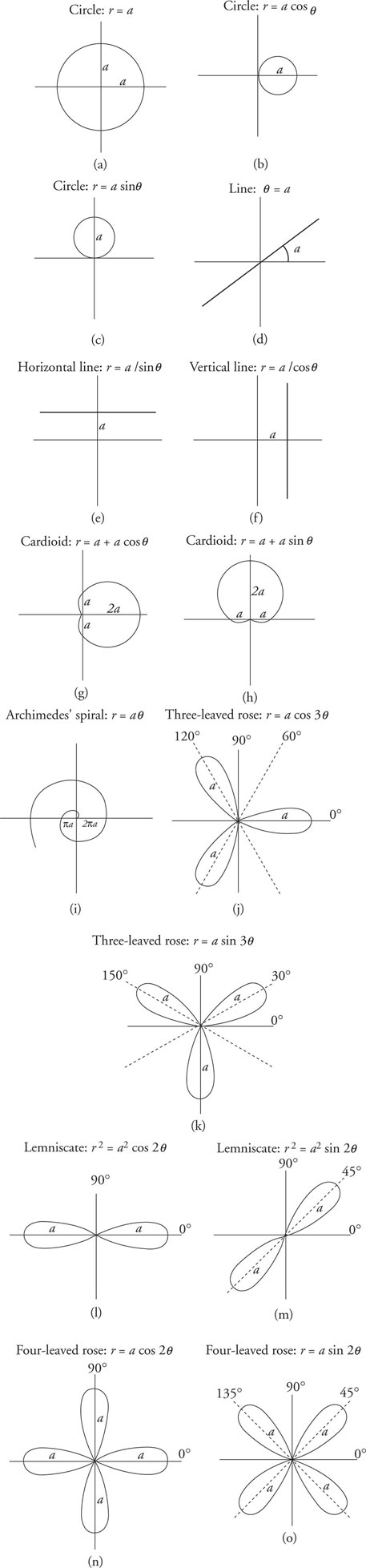## Polar Coordinates

Many systems and styles of measure are in common use today. When graphing on a flat surface, the rectangular coordinate system and the polar coordinate system are the two most popular methods for drawing the graphs of relations. Polar coordinates are best used when periodic functions are considered. Although either system can usually be used, polar coordinates are especially useful under certain conditions.

The rectangular coordinate system is the most widely used coordinate system. Second in importance is the polar coordinate system. It consists of a fixed point 0 called the pole, or origin. Extending from this point is a ray called the polar axis. This ray usually is situated horizontally and to the right of the pole. Any point, P, in the plane can be located by specifying an angle and a distance. The angle, θ, is measured from the polar axis to a line that passes through the point and the pole. If the angle is measured in a counterclockwise direction, the angle is positive. If the angle is measured in a clockwise direction, the angle is negative. The directed distance, r, is measured from the pole to point P. If point P is on the terminal side of angle θ, then the value of r is positive. If point P is on the opposite side of the pole, then the value of r is negative. The polar coordinates of a point can be written as an ordered pair ( r, θ). The location of a point can be named using many different pairs of polar coordinates. Figure illustrates three different sets of polar coordinates for the point P (4,50°).Figure 1
Polar forms of coterminal angles.

Conversion between polar coordinates and rectangular coordinates is illustrated as follows and in Figure  2.Figure 2
Polar to rectangular conversion.Example 1: Convert P(4,9) to polar coordinates.The polar coordinates for P (4, 9) are.

Example 2: Convert P (5,20°) to rectangular coordinates.The rectangular coordinates for P (5,20°) are P (4.7, 1.7).

Example 3: Transform the equation x 2 + y 2 + 5x = 0 to polar coordinate form.The equation r = 0 is the pole. Thus, keep only the other equation.Graphs of trigonometric functions in polar coordinates are very distinctive. In Figure 3 , several standard polar curves are illustrated. The variable a in the equations of these curves determines the size (scale) of the curve.Figure 3
Graphs of some common figures in polar form.